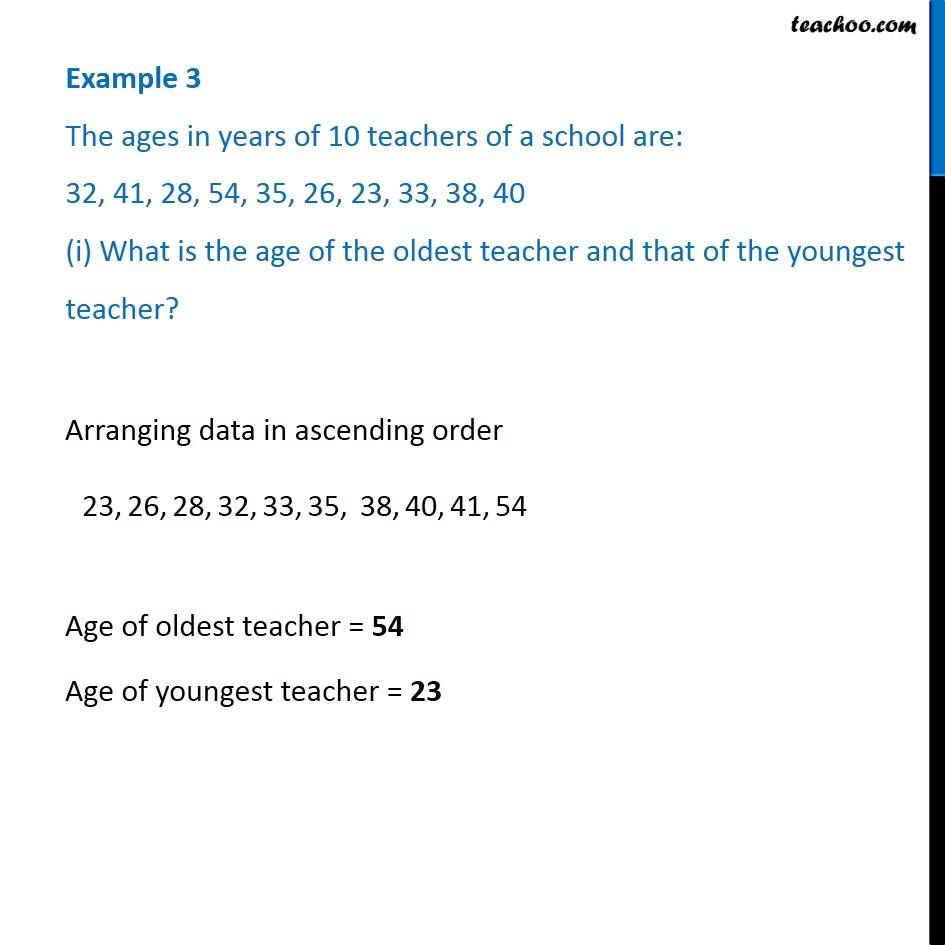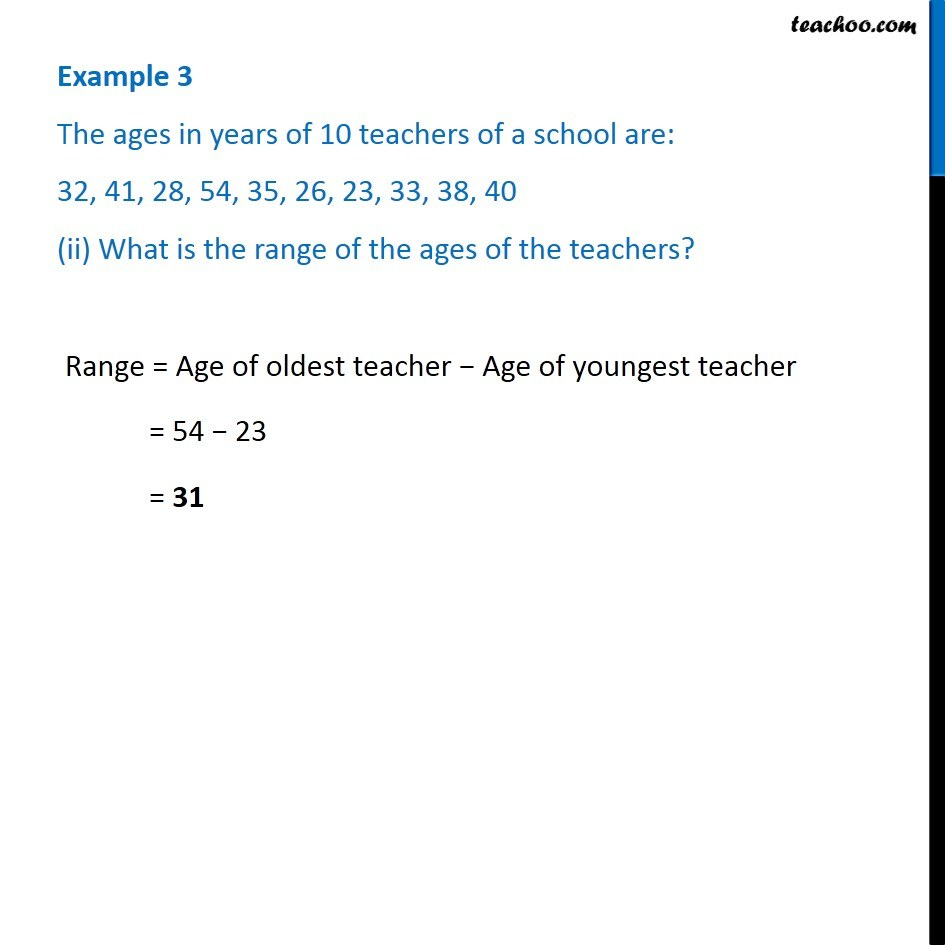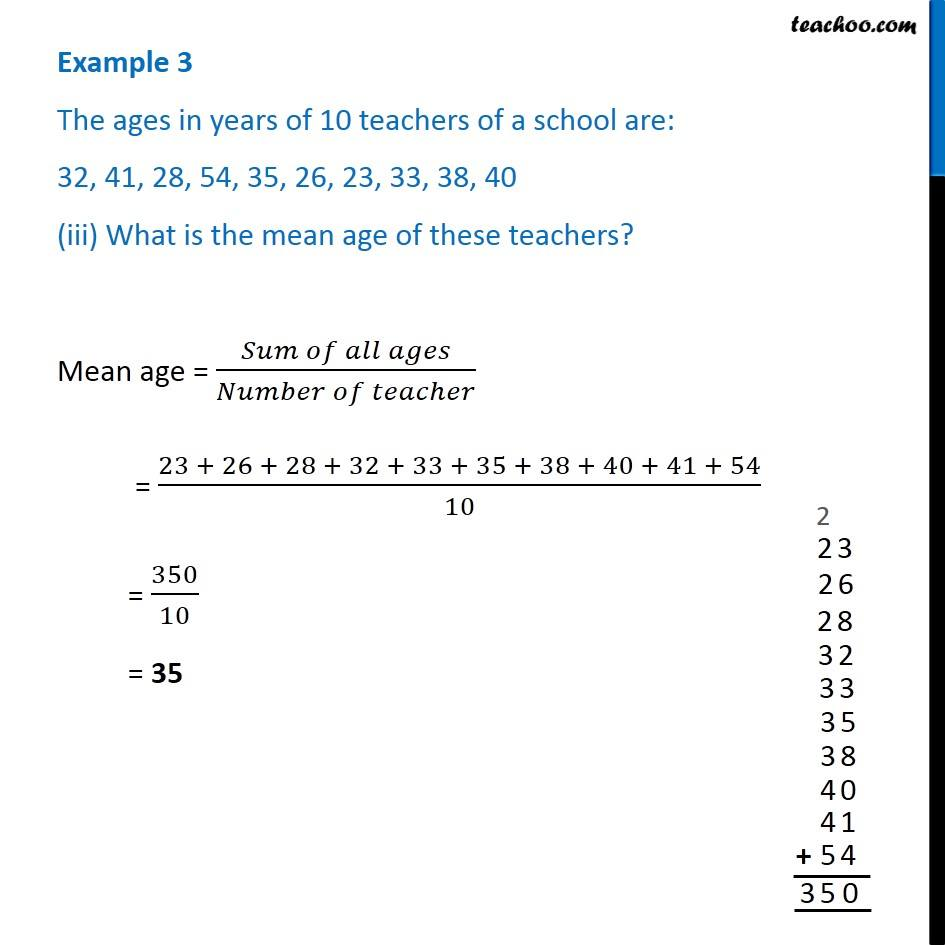Examples

Chapter 3 Class 7 Data Handling
Serial order wiseLearn in your speed, with individual attention - Teachoo Maths 1-on-1 Class

### Transcript

Example 3 The ages in years of 10 teachers of a school are: 32, 41, 28, 54, 35, 26, 23, 33, 38, 40 (i) What is the age of the oldest teacher and that of the youngest teacher? Arranging data in ascending order Age of oldest teacher = 54 Age of youngest teacher = 23 Example 3 The ages in years of 10 teachers of a school are: 32, 41, 28, 54, 35, 26, 23, 33, 38, 40 (ii) What is the range of the ages of the teachers? Range = Age of oldest teacher − Age of youngest teacher = 54 − 23 = 31 Example 3 The ages in years of 10 teachers of a school are: 32, 41, 28, 54, 35, 26, 23, 33, 38, 40 (iii) What is the mean age of these teachers? Mean age = (𝑆𝑢𝑚 𝑜𝑓 𝑎𝑙𝑙 𝑎𝑔𝑒𝑠)/(𝑁𝑢𝑚𝑏𝑒𝑟 𝑜𝑓 𝑡𝑒𝑎𝑐ℎ𝑒𝑟) = (23 + 26 + 28 + 32 + 33 + 35 + 38 + 40 + 41 + 54)/10 = 350/10 = 35